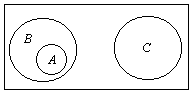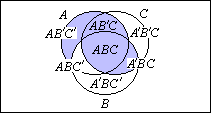# Controversial Venn Diagrams

Along with other tools - decision trees, graphs and truth tables, for example - Venn diagrams are used widely to bolster thought process in every walk of life, whenever decisions have to be made. Like L. Carroll's trilateral diagrams and Euler circles, Venn diagrams are helpful in solving puzzles, and also for validation and derivation of syllogisms. Deservedly, Venn diagrams are taught to young children and their teachers while being recommended to their parents, too.

When used without a proper definition, Venn diagrams are often confused with those of Euler that are more often referred to as Euler circles rather than Euler diagrams. The latter represent sets or arbitrary concepts exclusively as circles in a plane. Important in every specific case is the relative location of each of the circles. Manifestly, in Venn diagrams, sets are depicted by means of arbitrarily shaped (but bounded by simple closed curves) regions subject to a combinatorial requirement that, for a given number N of sets A1, ..., AN, there be exactly 2N connected subregions formed as intersections X1...XN, where each Xi is either Ai or its complement A'i. (Complements are taken with respect to a fixed universal set, say, U: X' = U\X.)

The most familiar Venn diagram depicts three sets (N = 3), bounded by three equal circles, as in the starting configuration of the two Java applets linked to below.

(Let me note in passing that the intersection of the three circles bears resemblance to the famous Reuleaux's triangle. In fact, the latter is obtained only in one case, where the circles pass through each other centers.)

Let's, for the sake of comparison, illustrate W. Dunham's syllogism both by means of Venn and Euler diagrams:

Euler circles Venn diagram All albatrosses are birds (region A). No camels are birds (region B for birds, region C for camels). No camels are albatrosses.A is entirely in B. C is altogether outside B. C is therefore outside A. AB'C' and AB'C are empty. ABC and A'BC are empty. Therefore, the non-empty part of C (A'B'C) is outside of the non-empty part of A (ABC').

The Venn diagram may look a little complicated, because of my attempt to identify all the regions (with the exception of A'B'C') involved. This is usually not necessary, see for example K. Devlin's treatment. In any event, Venn diagrams are judged to be more versatile than Euler circles.

Three circles in Venn diagrams split the universal set into 8 regions. Four circles, however positioned, may only create 14 regions at most. This is because any two circles intersect in at most 2 points. So that the fourth circle may only intersect the other three in 6 points. Thus the addition of a fourth circle to a 3-circle diagram can represent not more than 14 (= 8 + 6) regions, whereas 16 = 24 are required by the definition. For this reason, Venn diagrams with more than 3 sets use ovals, rounded rectangles, or other fancy shapes.

### References

1. S. Beckman, Mathematics for Elementary Teachers, Addison-Wesley, 2003
2. C. D. Miller, V. E. Heeren, J. Hornsby, Mathematical Ideas, 9th edition, Addison Wesley Longman, 2001
3. N. Rosenberg, How to Enjoy Mathematics with Your Child, Stein and Day, 1970
4. I. Stewart, Another Fine Math You've Got Me Into..., W. H. Freeman and Co, 1992### Lewis Carroll